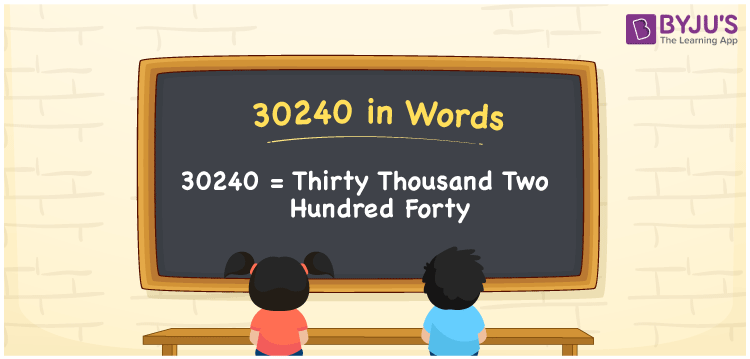# 30240 in Words

30240 in words is written as Thirty thousand two hundred forty. In both the International System of Numerals and the Indian System of Numerals, 30240 in words is written as Thirty thousand two hundred forty. The number 30240 is a Cardinal Number as it represents some quantity. For example, “she has 30240 rupees in her bank account”.

 30240 in Words Thirty thousand two hundred forty Thirty thousand two hundred forty in number 30240

## 30240 in English Words

30240 in English words is read as “Thirty thousand two hundred forty”.## How to Write 30240 in Words?

To write 30240 in words, we shall use the place value chart. In the place value chart, put 3 in the ten thousands, 0 in the thousands, 2 in the hundreds, 4 in the tens and 0 in the ones, respectively. Let us make a place value chart to write the number 30240 in words.

 Ten Thousands Thousands Hundreds Tens Ones 3 0 2 4 0

Thus, we can write the expanded form as

3 × Ten Thousand + 0 × Thousand + 2 × Hundred + 4 × Ten + 0 × One

= 3 × 10000 + 0 × 1000 + 2 × 100 + 4 × 10 + 0 × 1

= 30000 + 0 + 200 + 40 + 0

= 30240

= Thirty thousand two hundred forty.

30240 is a natural number that lies between 30239 and 30241.

30240 in words – Thirty thousand two hundred forty

• Is 30240 an odd number? – No
• Is 30240 an even number? – Yes
• Is 30240 a perfect square number? – No
• Is 30240 a perfect cube number? – No
• Is 30240 a prime number? – No
• Is 30240 a composite number? – Yes

## Frequently Asked Questions on 30240 in Words

Q1

### How to write 30240 in words?

30240 in words is written as Thirty thousand two hundred forty.
Q2

### How to write 30240 in the International and Indian System of Numerals?

In both, the system of numerals, 30240 in words, is written as Thirty thousand two hundred forty.
Q3

### How to write 30240 in a place value chart?

In the place value chart, write 3 in the ten thousands, 0 in the thousands, 2 in the hundreds, 4 in the tens and 0 in the ones, respectively.